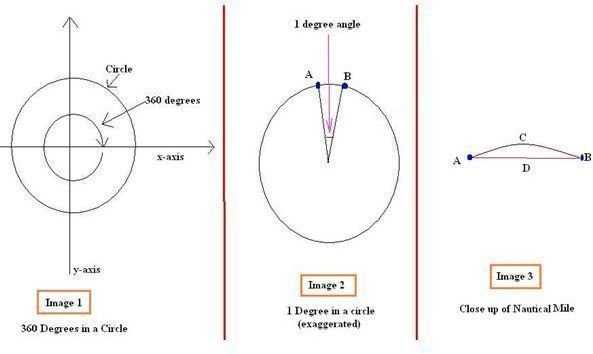How long is a nautical mile – nautical miles to kilometres

Introduction

We are all familiar with the units of length either in kilometers or miles and also know the relation between these two commonly used units. However people at sea are a bit different and hence do not use these measures but use a different unit of length which is known as the nautical mile. Also merchant ships do not boast of high speeds unless of course we are talking about special racing vessels such as the F1 Powerboat, hence the units for speed are different than land as seen below.

The Nautical Mile and Knot

Speaking in the simplest of terms a nautical mile is the distance equivalent to an arc of one minute on the surface of the earth. If you are getting confused by this simple definition perhaps you need to clear some of your doubts by reading the basic concepts below.

We all know from school level maths that a circle consists of 360 degrees of angle. If you divide the circle into 360 degrees it will look something like the wheel of your bicycle having spokes which are at a distance of one degree angle. Each of these degrees can be subdivided into sixty subdivisions each and they are known as minutes (don’t confuse with the unit of time).Take a look at the picture above to understand this. The first picture on the left side shows a normal circle which represents the Earth and the angle of the circle is 360 degrees. The circumference of this circle represents the circumference of the Earth. Now if this circle is divided into 360 equal parts and each part into 60 parts then one of those parts would look like what is shown in the middle picture. Of course this is not shown to scale otherwise you would perhaps need a magnifying glass to view it.

The third picture of the right shows even closer view of the minute arc. The blue dots represent the points A and B respectively which are the end points of the arc. Now the distance between A and B can be measured in two ways as shown in this third picture.

The first is the shortest or straight line distance between them and is represented by ADB while the other is the actual distance which a ship would have to travel because of the shape of the Earth and is represented by ACB. Now this ACB is the nautical mile and if you want to convert it into kilometers the formula for that is

One nautical mile = 1.852 kilometers

Hence we see that a nautical mile is nearly double than the normal kilometers used on road. The speed of a ship is hence expressed in terms of nautical miles per hour and this is known as knots for short.

Hence one knot ship speed = one nautical mile per hour speed or 1.852 km/hr.

How did we get this number?

If you want to know how we arrived at this number then let me tell you a simple mathematical formula which says that

Length of the Arc = A * pi/180 * r

• Where A is the angle in degrees which is 1/60 degrees in this case
• pi is constant and is given by 22/7
• r is radius of the Earth which is approximately 6378 kms

Hence if you put these figures in the formula you would get the value of the nautical mile